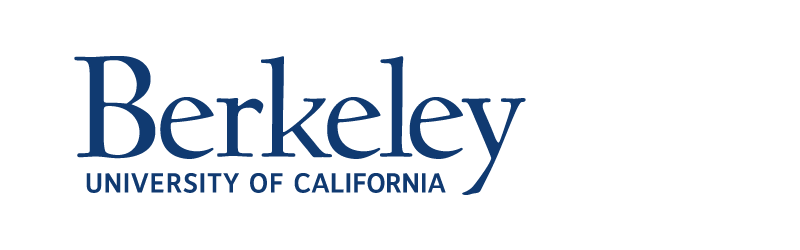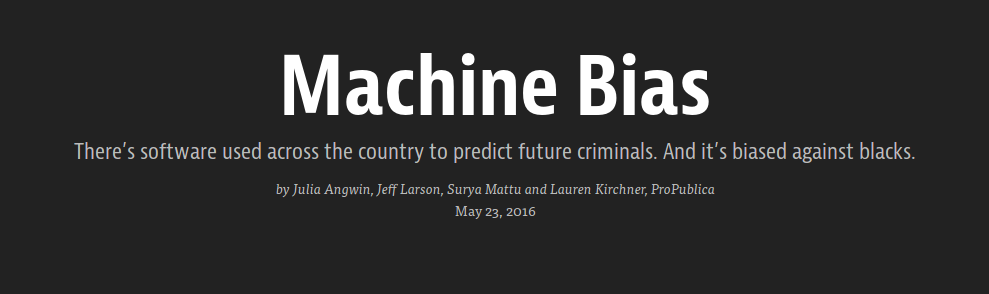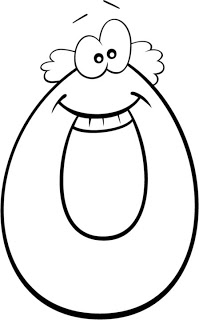# CS 294: Fairness in Machine Learning

## Moritz HardtSeptember 8, 2017

## Observational fairness criteria

Many definitions

Algorithms for achieving them

Impossibility results

}
Today!

## Typical setup

$X$ features of an individual

$A$ sensitive attribute (race, gender, ...)

$C=C(X,A)$ classifier mapping $X$ and $A$ to some prediction

$Y$ actual outcome

Note: random variables in the same probability space

Notation: $\mathbb{P}_a\{E\}=\mathbb{P}\{E\mid A=a\}.$

## All of this is a fragile abstraction

$X$ incorporates all sorts of measurement biases

$A$ often not even known, ill-defined, misreported, inferred

$C$ often not well defined, e.g., large production ML system

$Y$ often poor proxy of actual variable of interest

## Observational criteria

Definition.     A criterion is called observational if it is a property of the joint distribution of features $X,A$, classifier $C$, and outcome $Y$.

Anything you can write down as a probability statement involving $X, A, C, Y.$

What can we learn from observational criteria?

How can we achieve them algorithmically?

How do these criteria shape public discourses?

Key example: COMPAS debate on crime recidivism risk scores

## Demographic parity

Definition.     Classifier $C$ satisfies demographic parity if $C$ is independent of $A$.

When $C$ is binary $0/1$-variables, this means
$\mathbb{P}_a\{C = 1\} = \mathbb{P}_b\{C = 1\}$ for all groups $a,b.$

Approximate versions:

$$\frac{\mathbb{P}_a\{ C = 1 \}} {\mathbb{P}_b\{ C = 1 \}} \ge 1-\epsilon$$
$$\left|\mathbb{P}_a\{ C = 1 \}- \mathbb{P}_b\{ C = 1 \}\right|\le\epsilon$$

## Potential issues

### Lazyness

Accept random people in group $a$ but qualified people in group $b.$

Can happen for lack of training data (recall sample size disparity)

### Not optimality compatible

Rules out perfect classifier $C=Y$ when base rates are different: $\mathbb{P}_a\{C=1\}\ne\mathbb{P}_b\{C=1\}.$

Definition.     Classifier $C$ satisfies accuracy parity if
$\mathbb{P}_a\{ C = Y \} = \mathbb{P}_b\{ C = Y \}$ for all groups $a,b$.

### The good

Discourages laziness by equalizing error rates across groups.

Allows perfect predictor $C=Y.$

Error types matter!

Allows you to make up for rejecting qualified women by accepting unqualified men.

## True positive parity

Assume $C$ and $Y$ are binary $0/1$-variables.

Definition.     Classifier $C$ satisfies true positive parity if
$\mathbb{P}_a\{ C = 1 \mid Y=1\} = \mathbb{P}_b\{ C = 1\mid Y=1\}$ for all groups $a,b$.

Called equal opportunity in H-Price-Srebro (2016).

Suitable when positive outcome ($1$) is desirable.
Equivalently, primary harm is due to false negatives.

## False positive parity

Assume $C$ and $Y$ are binary $0/1$-variables.

Definition.     Classifier $C$ satisfies false positive parity if
$\mathbb{P}_a\{ C = 1 \mid Y=0\} = \mathbb{P}_b\{ C = 1\mid Y=0\}$ for all groups $a,b$.

TPP+FPP together called equalized odds in H-Price-Srebro (2016). We'll also call it positive rate parity.

In full generality, random variable $R$ satisifes equalized odds if $R$ is conditionally independent of $A$ given $Y.$

## Predictive value parity

Assume $C$ and $Y$ are binary $0/1$-variables.

Definition.     Classifier $C$ satisfies
• positive predictive value parity if for all groups $a,b$:
$\mathbb{P}_a\{ Y = 1 \mid C=1\} = \mathbb{P}_b\{Y = 1\mid C=1\}$
• negative predictive value parity if for all groups $a,b$:
$\mathbb{P}_a\{ Y = 1 \mid C=0\} = \mathbb{P}_b\{Y = 1\mid C=0\}$
• predictive value parity if it satisfies both of the above.

## Why predictive value parity?

Equalizes chance of success given acceptance.

## COMPAS: An observational debate## COMPAS: An observational debate

Probublica's main charge was observational.

Black defendants experienced higher false positive rate.

Northpointe's main defense was observational.

Scores satisfy precision parity.

Proposition.    Assume differing base rates and an imperfect classifier $C\ne Y.$ Then, either
• positive rate parity fails, or
• predictive value parity fails.

Observed in very similar form by Chouldechova (2017).

Similar trade-off result for score functions due to Kleinberg, Mullainathan, Raghavan (2016)

## Intuition

 Group a b OutcomeUnequal base rates PredictorSo far, predictor is perfect.

Let's introduce an error.

## Intuition

 Group a b OutcomeUnequal base rates PredictorBut this doesn't satisfy false positive parity!

Let's fix that!

## Intuition

 Group a b OutcomeUnequal base rates PredictorSatisfies positive rate parity!

## Intuition

 Group a b OutcomeUnequal base rates PredictorNPV 2/5 1/3

Does not satisfy predictive value parity!

Proof.    Assume unequal base rates $p_a, a\in\{0, 1\}$, imperfect classifier $C\ne Y$, and positive rate parity. W.l.o.g., $p_0>0$ (since $p_0=p_1=0$ is trivial)
Show that predictive value parity fails.

Proof by googling the first Wiki entry on this:

$\mathrm{PPV_a} = \frac{\mathrm{TPR}p_a}{\mathrm{TPR}p_a+\mathrm{FPR}(1-p_a)}$

Hence, $\mathrm{PPV}_0=\mathrm{PPV}_1$ implies
either $\mathrm{TPR}=0$ or $\mathrm{FPR}=0.$
(But not both, since $C\ne Y$)

$\mathrm{NPV_a} = \frac{(1-\mathrm{FPR})(1-p_a)}{(1-\mathrm{TPR})p_a+(1-\mathrm{FPR})(1-p_a)}$

In either case, $\mathrm{NPV}_0\ne \mathrm{NPV}_1.$ Hence predictive value parity fails.

## Score functions

Formally, any real-valued random variable $R\in[0,1]$ in the same probability space as $(X,A,Y)$.

Leads to family of binary classifiers by thresholding $C=\mathbb{I}\{R>t\}, t\in[0,1]$.

Different thresholds give different trade-offs between true and false positive rate.

## Bayes optimal scores

Goal: Find $R$ that minimizes $\mathbb{E}(Y-R(X,A))^2$

Solution: $R=r(X, A),$ where $r(x, a)=\mathbb{E}[Y\mid X=x, A=a].$

Given score $R$, plot (TPR, FPR) for all possible thresholds## Deriving classifiers from scores

Definition.   A classifier $C$ is derived from a score function $R$ if $C=F(R, A)$ where $F$ is a possibly randomized function.

Given score $R$ and cost $c=(c_{fn}, c_{fp}),$
derive classifier $C=F(R, A)$ that minimizes cost

• subject to no constraints.
• subject to equalized odds.
• subject to equality of opportunity.

## Deriving classifiers from scores

Construction on the board. See paper for details.

## Optimality preservation

An optimal equalized odds classifier can be derived
from the Bayes optimal regressor.

Theorem.    Let $R^*=\mathbb{E}[Y\mid X, A]$ be the Bayes optimal unconstrained score and let $c$ be a cost function for true and false positives.
There exists a derived equalized odds classifier $Y^*=F(R^*, A)$ that has minimal cost among all equalized odds classifiers.

Note: Approximate version of the theorem holds as well. See paper.

## FICO credit scores

Based on sample of 301536 TransUnion TransRisk scores from 2003
(made available by US Federal Reserve)

$R$ — Credit score ranging from 300 to 850

$Y$ — Default
Fineprint: failed to pay debt for at least $90$ days on at least one account

$A$ — Race (Asian, Black, Hispanic, White)

## FICO base rates## FICO score ROC curves by group## FICO score targets